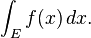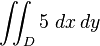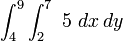# MULTIPLE INTEGRATION

Integrals can be taken over regions other than intervals. In general, an integral over a set E of a function f is written:Here x need not be a real number, but can be another suitable quantity, for instance, a vector in R3. Fubini’s theorem shows that such integrals can be rewritten as an iterated integral. In other words, the integral can be calculated by integrating one coordinate at a time.

Just as the definite integral of a positive function of one variable represents the area of the region between the graph of the function and the x-axis, the double integral of a positive function of two variables represents the volume of the region between the surface defined by the function and the plane which contains its domain. (The same volume can be obtained via the triple integral — the integral of a function in three variables — of the constant function f(x, y, z) = 1 over the above mentioned region between the surface and the plane.) If the number of variables is higher, then the integral represents a hypervolume, a volume of a solid of more than three dimensions that cannot be graphed.

For example, the volume of the cuboid of sides 4 × 6 × 5 may be obtained in two ways:

• By the double integralof the function f(x, y) = 5 calculated in the region D in the xy-plane which is the base of the cuboid. For example, if a rectangular base of such a cuboid is given via the xy inequalities 2 ≤ x ≤ 7, 4 ≤ y ≤ 9, our above double integral now readsFrom here, integration is conducted with respect to either x or y first; in this example, integration is first done with respect to x as the interval corresponding to x is the inner integral. Once the first integration is completed via the F(b) − F(a) method or otherwise, the result is again integrated with respect to the other variable. The result will equate to the volume under the surface.
• By the triple integralof the constant function 1 calculated on the cuboid itself.Welcome to my blog. My name is Nico. Admin of this blog. I am a student majoring in mathematics who dreams of becoming a professor of mathematics. I live in Kwadungan, Ngawi, East Java. Hopefully in all the posts I can make a good learning material to the intellectual life of the nation. After the read, leave a comment. I always accept criticism suggestion to build a better me again .. Thanks for visiting .. : mrgreen:

Posted on September 3, 2011, in education and tagged , , , , , , , . Bookmark the permalink. 2 Comments.

1.pututik
2.abedsaragih